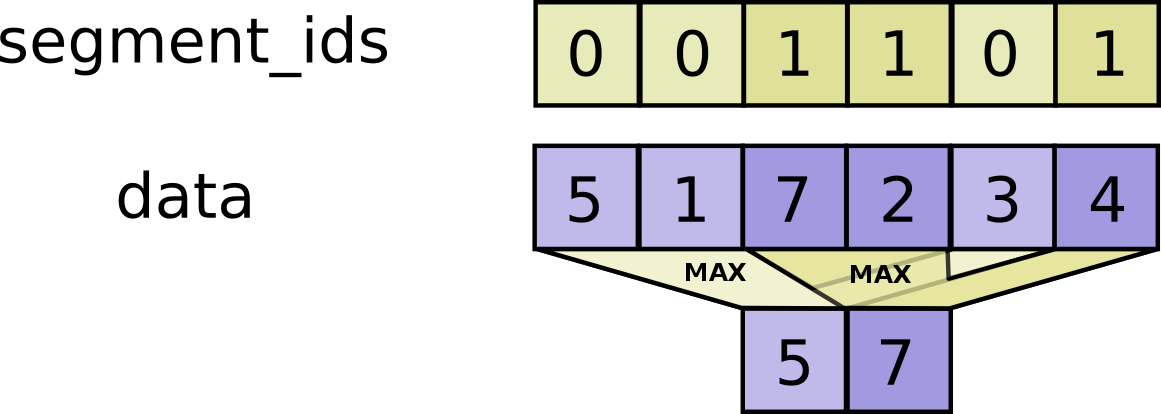# tf.math.unsorted_segment_max

Computes the maximum along segments of a tensor.

tf.math.unsorted_segment_max(
data, segment_ids, num_segments, name=None
)


Read the section on segmentation for an explanation of segments.

This operator is similar to the unsorted segment sum operator found (here). Instead of computing the sum over segments, it computes the maximum such that:

$$output_i = \max_{j...} data[j...]$$ where max is over tuples j... such that segment_ids[j...] == i.

If the maximum is empty for a given segment ID i, it outputs the smallest possible value for the specific numeric type, output[i] = numeric_limits<T>::lowest().

If the given segment ID i is negative, then the corresponding value is dropped, and will not be included in the result.#### For example:

c = tf.constant([[1,2,3,4], [5,6,7,8], [4,3,2,1]])
tf.unsorted_segment_max(c, tf.constant([0, 1, 0]), num_segments=2)
# ==> [[ 4,  3, 3, 4],
#       [5,  6, 7, 8]]


#### Args:

• data: A Tensor. Must be one of the following types: float32, float64, int32, uint8, int16, int8, int64, bfloat16, uint16, half, uint32, uint64.
• segment_ids: A Tensor. Must be one of the following types: int32, int64. A tensor whose shape is a prefix of data.shape.
• num_segments: A Tensor. Must be one of the following types: int32, int64.
• name: A name for the operation (optional).

#### Returns:

A Tensor. Has the same type as data.